2.16 Cyclic Symmetry:

 Observe the expression a+b+c --à(1) In the above expression, suppose we change a to b, b to c and c to a., what is the new expression? It is b+c+a -----à(2) Again, suppose we change b to c, c to a and a to b, what is the new expression? It is c+a+b  ---à(3)   We note that the expressions (1), (2) and (3) are all same.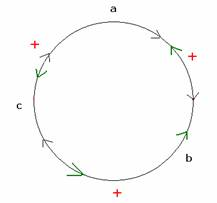Observe the expression a-b+c --à(1)

In the above expression, suppose we change a to b, b to c and c to a., what is the new expression?

It is b-c+a -----à(2)

Again, suppose we change b to c, c to a and a to b, what is the new expression?

It is c-a+b  ---à(3)

We note that the expressions (1), (2) and (3) are not same unless a=b=c

An expression f(x,y,z) is said to be cyclic with respect to x,y,z if f(x,y,z) = f(y,z,x) = f(z,x,y)

You will  again notice that the expressions x2+y2+ z2 and x3+y3 +z3 are cyclic with respect to variables x, y, z

The expression a+b+c is symbolically represented by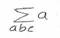Similarly the expression x3+y3 +z3 is symbolically represented by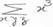2.16 Example 1: Write a2+b2+ c2-ab-bc-ca  using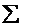notation

a2+b2+ c2-ab-bc-ca  = (a2+b2+ c2)-(ab+bc+ca)  =      (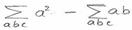)or =(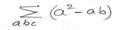)

2.16 Example 2:Expand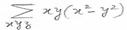= xy(x2-y2 )+yz(y2-z2)+zx(z2-x2)

2.16 Summary of learning

 No Points studied 1 Understanding of cyclic symmetry

Factorisation of Cyclic expressions:

2.16 Problem 1: Factorise ab(a-b)+bc(b-c)+ca(c-a)

We use the following identities for factorisation

 No Formula/Identity Expansion 1 (a+b)2 a2+b2+2ab 2 (a-b)2 a2+b2-2ab 3 (a+b)(a-b) a2-b2 4 (a+b)3 a3+b3+3ab(a+b) 5 (a-b)3 a3-b3-3ab(a-b) 6 a3+b3 (a+b) (a2 +b2 -ab) 7 a3-b3 (a-b) (a2 +b2 +ab)

Solution:

ab(a-b)+bc(b-c)+ca(c-a) = a2b - ab2+bc(b-c)+ c2a - ca2 (Expand first and last two terms)

= a2(b-c) - a(b2- c2) + bc(b-c) (Group those terms containing a2 and a together)

= a2(b-c) - a(b+c)(b-c) + bc(b-c) (Factorise (b2- c2))

= (b-c)(a2-a(b+c)+bc) (Taking out (b-c) as common factor)

= (b-c)(a2-ab-ac+bc) (Group those terms containing a and c together)

= (b-c)(a(a-b)-c(a-b))

= (b-c)((a-b)(a-c)

= - (a-b)(b-c)(c-a)

If all the terms of an algebraic expression are of the same degree then such an expression is called a Homogenous expression.

Ex. a2+b2+2ab is a homogenous expression.

a2+b2+a  is not a homogenous expression.